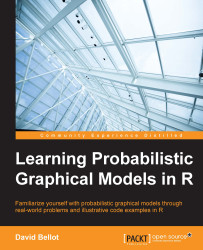•#### Learning Probabilistic Graphical Models in R#### Overview of this book

Probabilistic graphical models (PGM, also known as graphical models) are a marriage between probability theory and graph theory. Generally, PGMs use a graph-based representation. Two branches of graphical representations of distributions are commonly used, namely Bayesian networks and Markov networks. R has many packages to implement graphical models. We’ll start by showing you how to transform a classical statistical model into a modern PGM and then look at how to do exact inference in graphical models. Proceeding, we’ll introduce you to many modern R packages that will help you to perform inference on the models. We will then run a Bayesian linear regression and you’ll see the advantage of going probabilistic when you want to do prediction. Next, you’ll master using R packages and implementing its techniques. Finally, you’ll be presented with machine learning applications that have a direct impact in many fields. Here, we’ll cover clustering and the discovery of hidden information in big data, as well as two important methods, PCA and ICA, to reduce the size of big problems.
Learning Probabilistic Graphical Models in RCreditswww.PacktPub.comPrefaceFree Chapter
Probabilistic ReasoningExact InferenceLearning ParametersBayesian Modeling – Basic ModelsApproximate InferenceBayesian Modeling – Linear ModelsProbabilistic Mixture ModelsAppendixIndex## Chapter 4. Bayesian Modeling – Basic Models

After learning how to represent graphical models, how to compute posterior distributions, how to use parameters with maximum likelihood estimation, and even how to learn the same models when data is missing and variables are hidden, we are going to delve into the problem of modeling using the Bayesian paradigm. In this chapter, we will see that some simple problems are not easy to model and compute and will necessitate specific solutions. First of all, inference is a difficult problem and the junction tree algorithm only solves specific problems. Second, the representation of the models has so far been based on discrete variables.

In this chapter we will introduce simple, yet powerful, Bayesian models, and show how to represent them as probabilistic graphical models. We will see how their parameters can be learned efficiently, by using different techniques, and also how to perform inference on those models in the most efficient way. The algorithms...